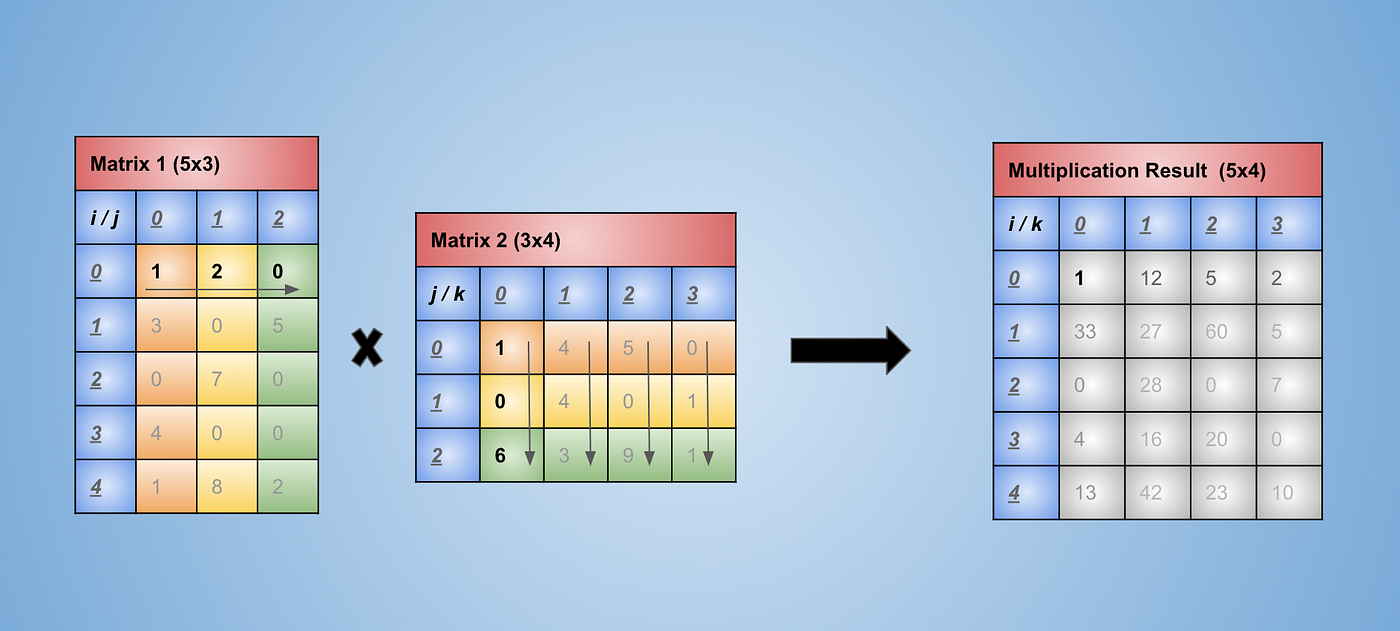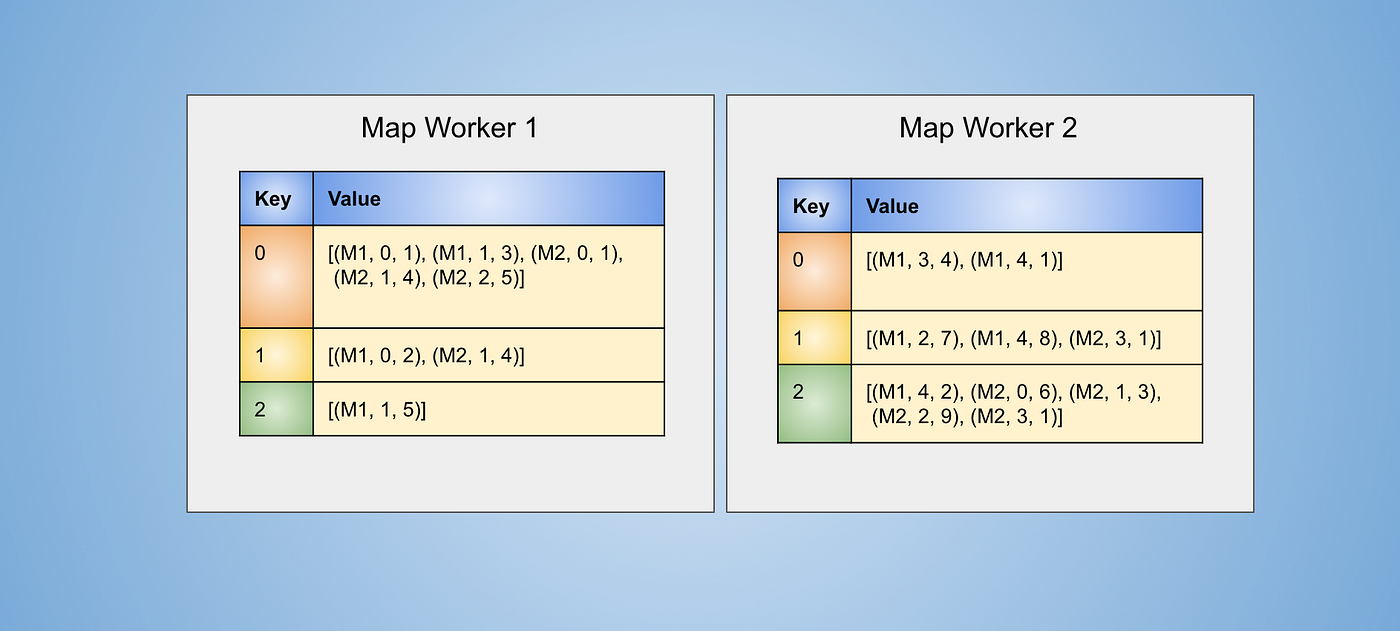# Matrix Multiplication At Scale Using Map Reduce

Matrix multiplication is the one of the most fundamental operation that most of the machine learning algorithms rely on. Knowing the working of matrix multiplication in a distributed system provides important insights on understanding the cost of our algorithms. Google’s PageRank algorithm is also based on repeated multiplication of matrices (matrix and a vector) to reach convergence (big sparse matrices). In this article we will understand how map reduce is used to multiply matrices that are so big that those don’t even fit on a single machine. The ideas used in this article are also extended to the algorithms that are used in GPUs to multiply matrices in parallel very efficiently. This is the third post in the series to understand map reduce, in case you are not familiar with working of map reduce please checkout the following two articles:

# Contents

• Matrix Representation
• Matrix Multiplication Using Two Passes
• Conclusion
• Code Snippet For Sparse Matrix Multiplication
• Resources

# Matrix Representation

To represent matrix we will be using COOrdinate format. We only store indices of the matrix that have non zero values and the value associated with that location. The following diagram shows how matrix looks in its raw form.

By storing only the indices that have non zero values we also end up saving a lot of space in case matrices are sparse (which is the case where matrices become way too large). The following image shows the representation of the above two matrices (matrix 1 and matrix 2) using the representation discussed above.

# Matrix Multiplication Using Two Passes

Here two passes symbolises the fact that we will need two map reduce jobs to compute the matrix multiplication. Let’s first try to understand the steps taken to multiply matrices. This explanation will be referred while explaining the operation in the passes.Elements involved of two matrices to get result for the first element

In above image we see that, to construct first element of result `1` in our case at position (0, 0) `(1 * 1) + (2 * 0) + (0 * 6) = 1`, we need to multiply the elements of first row of `matrix 1` with the elements of first column of the `matrix 2` . The colour signifies the elements that are multiplied together. The orange element is multiplied with orange element and the same for yellow and green elements.

If we follow the procedure of matrix multiplication we will see that all the orange elements in `matrix 1` needs to be multiplied with the orange elements of `matrix 2` and same for yellow and green ones.

To obtain value at an index `i, k` in the resultant matrix, we need to sum over the multiplication of the elements of `ith` row in matrix 1 and `kth` column in matrix 2.

Based on the above understanding we will design our first map reduce job to compute these multiplications. And the second job will be responsible to compute the sums.

Lets first take a look on how the data looks at the Map Workers (we will consider having 2 map workers and 2 reduce workers) when it is stored as the representation discussed in the previous section.

The above represented matrices can be seen as two relational tables with columns (i, j, v) and (j, k, v). Matrix multiplication does resemble a lot to a natural join over the `j` column, followed by a sum aggregation.

• Map Function Pass 1: We want to achieve the same key for all the orange elements in the matrix used for explanation and same for yellow and green coloured elements, so that we can then take all the values and multiply those together to form the partial multiplication results as discussed in the explanation above. For each row of matrix 1 create key-value pair of form `j:(M1, i, vij)` . Where `M1` represents that fact that this value came from matrix 1, and `vij` represents the value for row for given `i, j` values in the relation. For each row of matrix 2 create key-value pair of form `j: (M2, k, vjk)`. We need to keep track of from which matrix the value came from as we don’t want to multiply the elements of the same matrix.
• Reduce Function Pass 1: Once we have the same coloured elements of a matrix in one place we just need to multiply those and output the result in key-value form which can be fed to the next map reduce job. For a key `j` take each value that comes from M1 of form`(M1, i, vij)` and take each value that comes from M2 of the form `(M2, k, vjk)` and produce a key-value pair of form `i,k: vij * vjk`.

After application of map function and grouping of keys at map workers the data looks like the following figure, notice that each key has different number of values, this is the case because we don’t store data about the location where value is zeroData at Map workers after applying map function and grouping keys

Files for reduce workers will be created at the map workers, the following figures shows the content in those files

The files are sent to reduce workers where the files will be as follows:

After this we apply the reduce function which will generate intermediate output in this case for the next pass of map reduce. Which involves multiplication of the values which came from Matrix 1 with all the values that came from Matrix 2.

For example for `j` value `1` we generate the keys as follows

`Key: 1Index:  [    0            1           2           3           4    ]Values: [(M1, 0, 2), (M1, 2, 7), (M1, 4, 8), (M2, 1, 4), (M2, 3, 1)]For value at index 0, 1, 2 which are from matrix 1, we need to multiply the values from index 3 and 4 as those are from matrix 2Forming the key, values in yellow colour in the below imagee.g. the key (0, 1) for reducer worker 2 is formed by multiplying values from index 0 and index 3. Which are 2, 4 in this case resulting in output tuple {(0, 1): 8}.`

Now with the multiplication done and all we want to do is group by the key and apply sum aggregation and output data in the form `i, k, value` Where i, k are the indices of the resultant matrix and `value` is the value at those indices.

• Map Function Pass 2: Map function doesn’t need to do anything as we have the input in a key value form.
• Reduce Function Pass 2: Reduce function just needs to sum for values associated with the same key.

Assuming that data at reduce workers is sent back to map workers, we will have to create files for reduce workers to consume based on some hash function that make sure that same keys goes to one reduce worker. The files will look like:

The files are sent to reduce workers where these look like:

Finally reduce function is applied which adds up the values for a common key within all the files in reduce worker and output is generated in form `i, k, value`

Above is the result of matrix multiplication of the two matrices represented in COO format. This can be stored to files in some storage system if required.

# Conclusion

In this article we saw the nature of matrix multiplication to be really great for parallel processing, but also saw how it generated a few keys but a lot of values, this can be troublesome in case the matrix is huge and list of values become so huge that it doesn’t fit on a single machine. In such a case we can either increase the memory size of the worker nodes or even separate the matrix into small rectangular chunks, how that works is explained in section 5.2 of fifth chapter. This matrix multiplication can also be done in a single pass as explained in section 2.2.3 of second chapter. But on further reading we find out that the communication cost of the two pass algorithm is better than the one pass algorithm, making two pass algorithm much efficient in most cases, which is counter intuitive to most. In section 2.5 of second chapter the topic of communication cost is explained in detail and is helpful to understand the mathematical calculation which is used inside query optimizers to decide how to perform operations like joins and matrix multiplications based on a few data statistics.

# Code Snippet For Sparse Matrix Multiplication

I’ve created the following snippet to visualize output of the steps that will be created during map reduce steps above. I’ve done that using raw python code to make it possible to execute this for any matrix. Originally this code was written with the objective to multiply sparse matrices efficiently on a single system, but is generic for any matrix. There are no partitions or parallel operations here, this can be thought as a system with single map worker that is also used for the reduce worker.

--

--